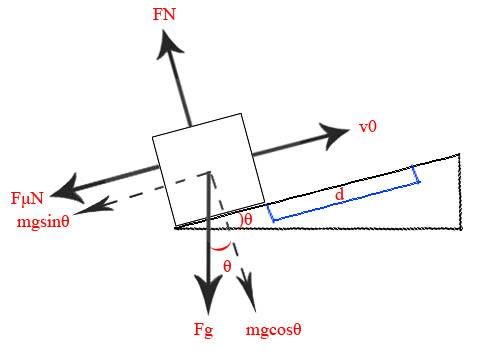# Classical block on inclined plane

• cassinova
In summary, the homework statement is that a block at t=0 is at the bottom of the plane and is projected up an inclined plane with initial speed v0. The plane's acute angle is θ above the horizontal, and the coefficient of friction is μ between the block and plane. Find the time (t) and velocity (v1) of the block when it reaches a given distance d.f

## Homework Statement

A block at t=0 is at the bottom of the plane and is projected up an inclined plane with initial speed v0. The plane's acute angle is θ above the horizontal, and the coefficient of friction is μ between the block and plane.

Find the time (t) and velocity (v1) of the block when it reaches a given distance d.

## Homework Equations

1. F=ma
2. d=(1/2)at^2 + v0t + d0
3. v1=at+v0

## The Attempt at a Solution

I drew a picture electronically:I made the direction FN and v0 positive.

#1
x dir
F = ma
F = -FμN - mgsinθ
ma = -μmgcosθ - mgsinθ
a = g(-μcosθ - sinθ)

So I found my a (should be a negative value), and now I want to find t when the block reaches d.

#2
d = (1/2)(-at^2) +v0t +d0
0 = (1/2)(-at^2) +v0t + 0 - d

Problem: Acceleration and distance are negative so the roots are imaginary, but I need to find real roots of t and then plug it in v1=at+v0 (I think).

Why do you have -a in equation #2?

And why do you think that the roots are imaginary? They depend on d; if it is sufficiently small, roots will be real. This makes perfect sense physically, because there is some maximum distance that the block can travel at any initial velocity.

Problem: Acceleration and distance are negative so the roots are imaginary, but I need to find real roots of t and then plug it in v1=at+v0 (I think).
That does not automatically make the roots imaginary. There will be real roots if v0 is nonzero and d is sufficiently small.

It's just that when I graph 0 = (1/2)(-at^2) +v0t + 0 - d, the entire curve is below the x-axis.

It's just that when I graph 0 = (1/2)(-at^2) +v0t + 0 - d, the entire curve is below the x-axis.

Consider ## a = 2, \ v_0 = 5, \ d = 4 ##: is your statement true?